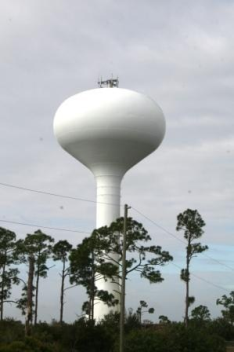# 2.2: Applying the Math of Geometric Shapes

$$\newcommand{\vecs}{\overset { \rightharpoonup} {\mathbf{#1}} }$$ $$\newcommand{\vecd}{\overset{-\!-\!\rightharpoonup}{\vphantom{a}\smash {#1}}}$$$$\newcommand{\id}{\mathrm{id}}$$ $$\newcommand{\Span}{\mathrm{span}}$$ $$\newcommand{\kernel}{\mathrm{null}\,}$$ $$\newcommand{\range}{\mathrm{range}\,}$$ $$\newcommand{\RealPart}{\mathrm{Re}}$$ $$\newcommand{\ImaginaryPart}{\mathrm{Im}}$$ $$\newcommand{\Argument}{\mathrm{Arg}}$$ $$\newcommand{\norm}{\| #1 \|}$$ $$\newcommand{\inner}{\langle #1, #2 \rangle}$$ $$\newcommand{\Span}{\mathrm{span}}$$ $$\newcommand{\id}{\mathrm{id}}$$ $$\newcommand{\Span}{\mathrm{span}}$$ $$\newcommand{\kernel}{\mathrm{null}\,}$$ $$\newcommand{\range}{\mathrm{range}\,}$$ $$\newcommand{\RealPart}{\mathrm{Re}}$$ $$\newcommand{\ImaginaryPart}{\mathrm{Im}}$$ $$\newcommand{\Argument}{\mathrm{Arg}}$$ $$\newcommand{\norm}{\| #1 \|}$$ $$\newcommand{\inner}{\langle #1, #2 \rangle}$$ $$\newcommand{\Span}{\mathrm{span}}$$$$\newcommand{\AA}{\unicode[.8,0]{x212B}}$$

As with all mathematical computations, there is an element of “Will I ever use this outside of the classroom?”. The answer is most likely “sometimes.” An operator might calculate the volume of water in a storage structure or pipeline to determine how much chlorine is needed to disinfect the structure. A contractor might calculate the internal surface area of a tank to determine the amount of coating that is required. Or, you might be asked to paint the interior walls of a room. Putting practical use to mathematical equations can help in the student’s overall understanding. The following problems are some “real-world examples” you might find working as a water utility operator.

## ExercisesFigure $$\PageIndex{1}$$This page titled 2.2: Applying the Math of Geometric Shapes is shared under a CC BY license and was authored, remixed, and/or curated by Mike Alvord (ZTC Textbooks) .